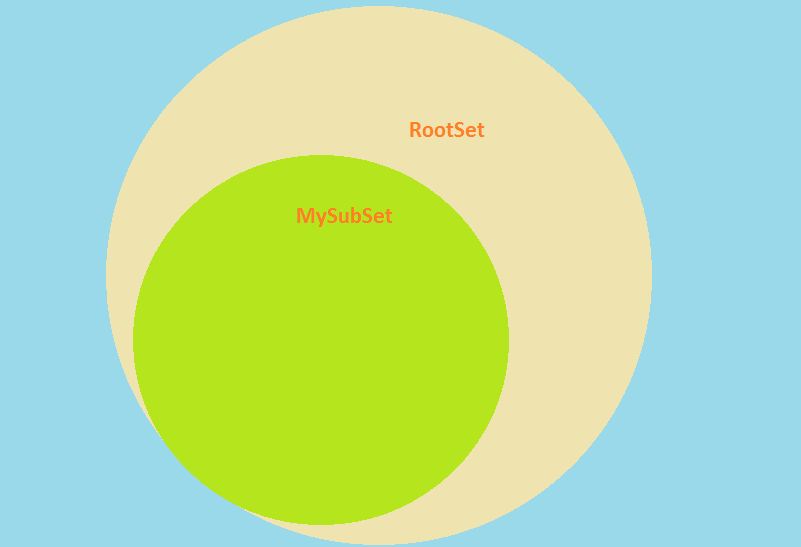# Local Binding vs. Default BindingIn this article we will compare two ways of defining index binding: local binding and default binding.

Assume there are two sets:

```1Set s_RootSet {
2    Index: i_rs;
3}
```

and

```1Set s_SubSet {
2    Index: i_ss;
3}
```

Let’s compare the expressions `( i in MySubSet )` and `( i \| i in MySubSet )`.

When you use `(i in MySubSet)`, AIMMS will handle `i` as an index in `s_Subset`. We say `i` is used as local binding in this case.

When you write `(i \| i in MySubSet)`, AIMMS will still consider `i` as an index of its original set `s_RootSet`. We say `i` is used as default binding in this case.

In general, using local binding and default binding doesn’t make a difference, but when element ordering is used, such as, `ord(i)`, `i+1` and `i–1`, it will have an impact. For instance, the following statement:

```Parameter1(i in MySubSet) := ord(i);
```

is the same as

```Parameter1(i | i in MySubSet) := ord(i,MySubSet );
```

while

```Parameter1(i | i in MySubSet) := ord(i);
```

is the same as

```Parameter1(i | i in MySubSet) := ord(i,RootSet );
```

So there is a difference between the two only if you use certain functions that are relative to the set. A complete explanation can be found in the section “Index Binding” of AIMMS Language Reference.